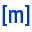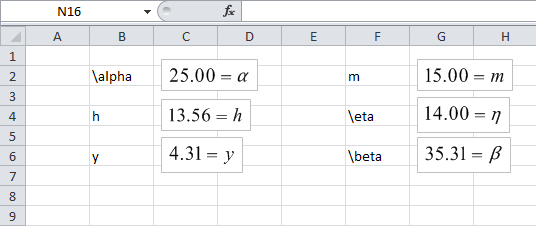## Display Units

Adds or removes units from the rendered equation. The units are taken from the cell immediately to the right of the one being rendered, or from cells referenced by the formula. If no related rendered equation exists, this button will render the selected cell and equate it to the content of the cell immediately to its left.

### Appearance

The units are displaced to the right of the endered equation in square brackets. The units are always assumed to be defined in LaTeX notations.

### Usage

• If no units are provided for the selected cell, then fxRender will form an expression for the units using any available units associated with referenced cells - essentially displaying the rendered equation again but with units instead of variables used. This feature is perfect for checking dimension consistency of an equation.
• Display Unit can be used on its own to render a new equation, using the selected cell as the assignment, with the cell on the immediate left becoming the main equation. As an example:
• becomes:Style: Excel Buttons:
• Display Unit can also be configured as a default option in all rendered equations. To change the default state, either click the button when an empty cell is selected, or click it with control-key selected.

### Example 7

Download the example provided and follow the steps below:

• To obtain units, fxRender looks at the cell immediately to the right of the cell containing a formula.
•Example 7: Style: Excel Buttons:
• LaTeX commands can be used to form these units. For example:
• LaTeX Units Displayed
N/mm^2
\frac{N}{mm^2}